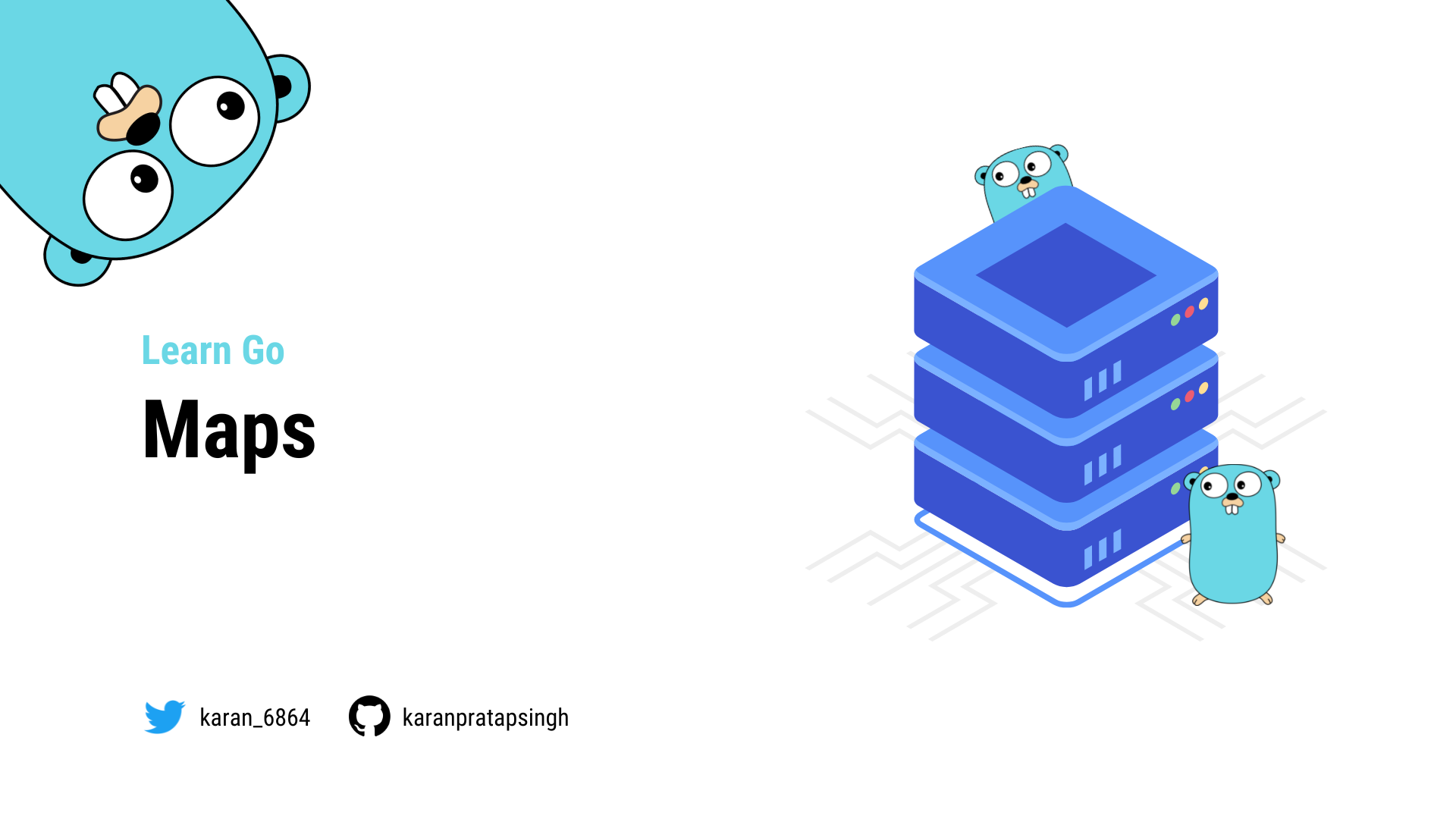# Maps

Authors
•Name
Karan Pratap Singh
@karan_6864So, Go provides a built-in map type, and we'll learn how to use it.

But, the question is what are maps? And why do we need them?Well, A map is an unordered collection of key-value pairs. It maps keys to values. The keys are unique within a map while the values may not be.

It is used for fast lookups, retrieval, and deletion of data based on keys. It is one of the most used data structures.

## Declaration

A map is declared using the following syntax:

``````var m map[K]V
``````

Where `K` is the key type and `V` is the value type.

For example, here's how we can declare a map of `string` keys to `int` values.

``````func main() {
var m map[string]int

fmt.Println(m)
}
``````
``````\$ go run main.go
nil
``````

As we can see, the zero value of a map is `nil`.

A `nil` map has no keys. Moreover, any attempt to add keys to a `nil` map will result in a runtime error.

## Initialization

There are multiple ways to initialize a map.

make function

We can use the built-in `make` function, which allocates memory for referenced data types and initializes their underlying data structures.

``````func main() {
var m = make(map[string]int)

fmt.Println(m)
}
``````
``````\$ go run main.go
map[]
``````

map literal

Another way is using a map literal.

``````func main() {
var m = map[string]int{
"a": 0,
"b": 1,
}

fmt.Println(m)
}
``````

Note that the trailing comma is required.

``````\$ go run main.go
map[a:0 b:1]
``````

As always, we can use our custom types as well.

``````type User struct {
Name string
}

func main() {
var m = map[string]User{
"a": User{"Peter"},
"b": User{"Seth"},
}

fmt.Println(m)
}
``````

We can even remove the value type and Go will figure it out!

``````var m = map[string]User{
"a": {"Peter"},
"b": {"Seth"},
}
``````
``````\$ go run main.go
map[a:{Peter} b:{Seth}]
``````

Now, let's see how we can add a value to our map.

``````func main() {
var m = map[string]User{
"a": {"Peter"},
"b": {"Seth"},
}

m["c"] = User{"Steve"}

fmt.Println(m)
}
``````
``````\$ go run main.go
map[a:{Peter} b:{Seth} c:{Steve}]
``````

## Retrieve

We can also retrieve our values from the map using the key.

``````...
c := m["c"]
fmt.Println("Key c:", c)
``````
``````\$ go run main.go
key c: {Steve}
``````

What if we use a key that is not present in the map?

``````...
d := m["d"]
fmt.Println("Key d:", d)
``````

Yes, you guessed it! we will get the zero value of the map's value type.

``````\$ go run main.go
Key c: {Steve}
Key d: {}
``````

## Exists

When you retrieve the value assigned to a given key, it returns an additional boolean value as well. The boolean variable will be `true` if the key exists, and `false` otherwise.

Let's try this in an example:

``````...
c, ok := m["c"]
fmt.Println("Key c:", c, ok)

d, ok := m["d"]
fmt.Println("Key d:", d, ok)
``````
``````\$ go run main.go
Key c: {Steve} Present: true
Key d: {} Present: false
``````

## Update

We can also update the value for a key by simply re-assigning a key.

``````...
m["a"] = "Roger"
``````
``````\$ go run main.go
map[a:{Roger} b:{Seth} c:{Steve}]
``````

## Delete

Or, we can delete the key using the built-in `delete` function.

Here's how the syntax looks:

``````...
delete(m, "a")
``````

The first argument is the map, and the second is the key we want to delete.

The `delete()` function doesn't return any value. Also, it doesn't do anything if the key doesn't exist in the map.

``````\$ go run main.go
map[a:{Roger} c:{Steve}]
``````

## Iteration

Similar to arrays or slices, we can iterate over maps with the `range` keyword.

``````package main

import "fmt"

func main() {
var m = map[string]User{
"a": {"Peter"},
"b": {"Seth"},
}

m["c"] = User{"Steve"}

for key, value := range m {
fmt.Println("Key: %s, Value: %v", key, value)
}
}
``````
``````\$ go run main.go
Key: c, Value: {Steve}
Key: a, Value: {Peter}
Key: b, Value: {Seth}
``````

Note that a map is an unordered collection, and therefore the iteration order of a map is not guaranteed to be the same every time we iterate over it.

## Properties

Lastly, let's talk about map properties.

Maps are reference types, which means when we assign a map to a new variable, they both refer to the same underlying data structure.

Therefore, changes done by one variable will be visible to the other.

``````package main

import "fmt"

type User struct {
Name string
}

func main() {
var m1 = map[string]User{
"a": {"Peter"},
"b": {"Seth"},
}

m2 := m1
m2["c"] = User{"Steve"}

fmt.Println(m1) // Output: map[a:{Peter} b:{Seth} c:{Steve}]
fmt.Println(m2) // Output: map[a:{Peter} b:{Seth} c:{Steve}]
}
``````